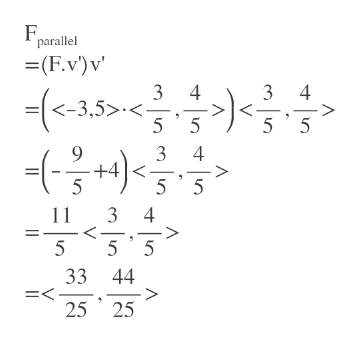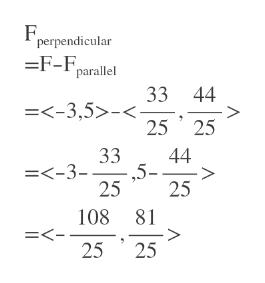Given vector ~v =< 3, 4 > and force vector F~ =< −3, 5 >, finda) The component of F~ parallel to ~v.b) The component of F~ perpendicular to ~v.c) The work, W, done by force F~ through displacement ~v.

Question

Given vector ~v =< 3, 4 > and force vector F~ =< −3, 5 >, find
a) The component of F~ parallel to ~v.

b) The component of F~ perpendicular to ~v.

c) The work, W, done by force F~ through displacement ~v.

Step 1

F~parallel = (F · v) v, where v is a unit vector pointing in the same direction as v.

||v||=sqrt(3^2+4^2)=sqrt(25)=5

unit vector in direction of v=v'=<3/5,4/5>

Step 2

F_parallel= <33/25 , 44/25>help_outlineImage Transcriptioncloseparallel (F.v)v 기를 - 3 4 3 4 =<-3,5>< 5 5 5 5 9 +4 5 3 4 5 5 11 3 4 < 5 5 5 33 44 =< 25 25 fullscreen
Step 3

F_perpendicular= F - F_parallelhelp_outlineImage Transcriptioncloseperpendicular =F-parallel 33 44 =<-3,5>-< 25 25 44 ,5- 25 33 =<-3- > 25 108 81 > 25 =<- 25 fullscreen

Want to see the full answer?

See Solution

Want to see this answer and more?

Our solutions are written by experts, many with advanced degrees, and available 24/7

See Solution
Tagged in

Other